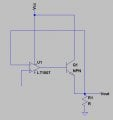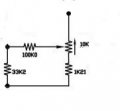# How does an op amp with negative feedback featuring a transistor work?

#### trherzfeld

Joined Jul 2, 2015
9
Hey, sorry for the basic question, but I was just curious on an op amp works when it feeds into a transistor and when it uses the emitter output as its negative feedback? Also, if R1 was the resistor/pot network seen, how would the pot affect the output? Any help/explanation is greatly appreciated, I'm just trying to understand how the circuit works. Thanks.#### AnalogKid

Joined Aug 1, 2013
10,791
Q1 is configured as an emitter follower. It has a voltage gain of 1 and is non-inverting, so it has very little effect on the workings of the negative feedback loop. It also has current gain, so where the opamp output might be able to source only 20 mA into R1, Q1 as a power transistor could source over one amp.

U1 is configured as a voltage follower, a non-inverting circuit with a gain of 1. This is used frequently to buffer a signal so that it can drive a larger load current. Basically, the voltage at the 1007 + input appears across R1 with much more current capability.

ak

#### WBahn

Joined Mar 31, 2012
29,519
Also, if R1 was the resistor/pot network seen, how would the pot affect the output?
If "the output" is Vout shown in the left diagram, then it would have little, if any, effect. Vout is going to be within microvolts (or perhaps a millivolt) of the voltage applied to the non-inverting input of the op-amp.

#### ian field

Joined Oct 27, 2012
6,536
Hey, sorry for the basic question, but I was just curious on an op amp works when it feeds into a transistor and when it uses the emitter output as its negative feedback? Also, if R1 was the resistor/pot network seen, how would the pot affect the output? Any help/explanation is greatly appreciated, I'm just trying to understand how the circuit works. Thanks.

View attachment 101239 View attachment 101240
Its just a voltage follower - much like if you just took the op-amp output back to the inverting input.

You lose the 0.7 Vbe from the available Vcc headroom, but it can source more current than the bare op-amp.

#### crutschow

Joined Mar 14, 2008
33,365
Basically the negative feedback means that the op amp will drive the base of the transistor such as to make the transistor emitter output equal the voltage applied to the op amp non-inverting (+) input.

The effect of the 0.7V base-emitter offset is reduced by the open-loop gain of the op amp so, for example, if the open-loop gain of the op amp was 100,000, then this would appear as 0.7V / 100,000 = 7μV offset in the output voltage (negligible for most applications).

•cmartinez

#### ian field

Joined Oct 27, 2012
6,536
Basically the negative feedback means that the op amp will drive the base of the transistor such as to make the transistor emitter output equal the voltage applied to the op amp non-inverting (+) input.

The effect of the 0.7V base-emitter offset is reduced by the open-loop gain of the op amp so, for example, if the open-loop gain of the op amp
But you still have to subtract it from the available Vcc headroom.

#### WBahn

Joined Mar 31, 2012
29,519
Basically the negative feedback means that the op amp will drive the base of the transistor such as to make the transistor emitter output equal the voltage applied to the op amp non-inverting (+) input.

The effect of the 0.7V base-emitter offset is reduced by the open-loop gain of the op amp so, for example, if the open-loop gain of the op amp was 100,000, then this would appear as 0.7V / 100,000 = 7μV offset in the output voltage (negligible for most applications).
Very true, but whatever output voltage you could achieve without the transistor (just the opamp) is now reduced by ~0.7 V -- that's the headroom that Ian was talking about.

#### dannyf

Joined Sep 13, 2015
2,197
The opamp adjust its output so that the output on the emitter equals that of the input.

R1s value, within reason, does not impact the output, if you follow the reasoning above.

#### crutschow

Joined Mar 14, 2008
33,365
But you still have to subtract it from the available Vcc headroom.
True. Never implied otherwise.